Function Repository Resource:

# EstimateSudokuDifficultyLevel

Estimate the level of difficulty for a given sudoku puzzle

Contributed by: Paritosh Mokhasi
 ResourceFunction["EstimateSudokuDifficultyLevel"][mat] estimates the difficulty level of a sudoku puzzle specified as mat.

## Details and Options

The input matrix must be a SparseArray square matrix.
ResourceFunction["EstimateSudokuDifficultyLevel"] returns a value between 1 and 10, with 1 being extremely easy and 10 being extremely hard.
The function estimates the difficulty level by recursively applying the standard rules of sudoku puzzles until no unique elements are left.
The three rules of a standard n×n sudoku board are:
First, each row must contain all the numbers 1–n.
Second, each column must contain all the numbers 1–n.
Third, each 3×3 block must contain all the numbers 1–n.
The function returns \$Failed if it finds that the sudoku puzzle violates any of the puzzle rules.

## Examples

### Basic Examples (2)

Estimate the difficulty level of the specified sudoku puzzle:

 In:=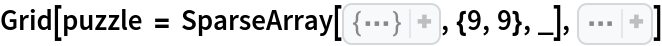Out=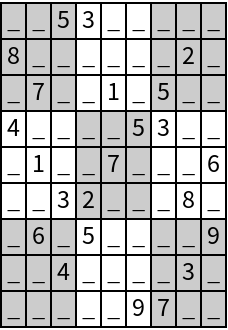In:=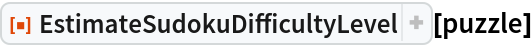Out=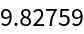Estimate the difficulty level of a 1616 sudoku puzzle:

 In:=Out=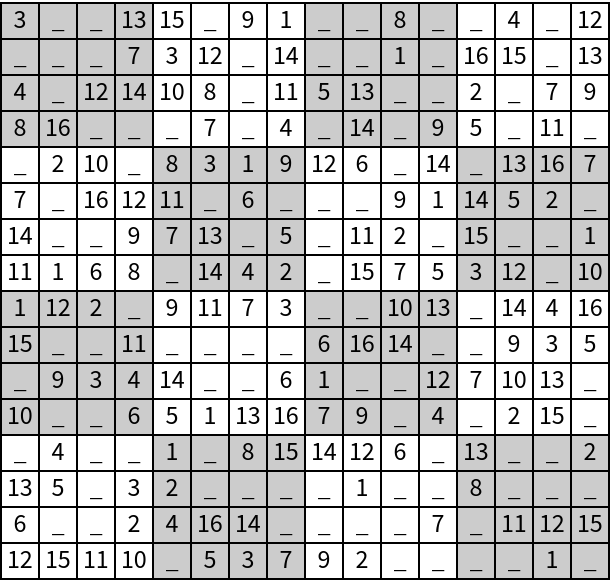In:=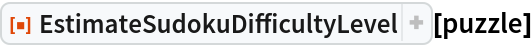Out=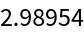Paritosh Mokhasi

## Version History

• 1.0.0 – 21 May 2020